### Home > CALC > Chapter 11 > Lesson 11.1.3 > Problem11-33

11-33.
1. Examine the integrals below. Consider the multiple tools available for integrating and use the best strategy. After evaluating each integral, write a short description of your method. Homework Help ✎

1.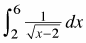2.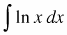3.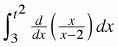4.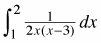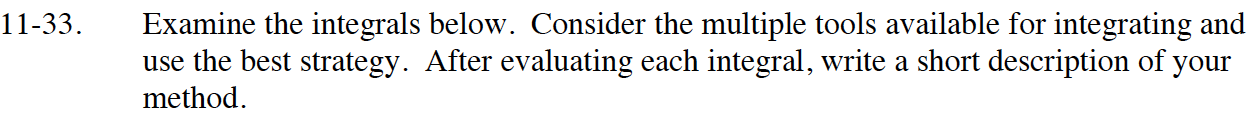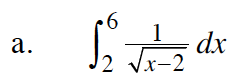$=\lim_{a\to 2}\int_a^6(x-2)^{-1/2}dx$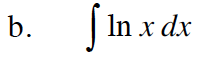Use integration by parts. Let f = ln(x) and dg = dx.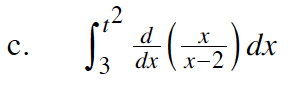This is the integral of a derivative, so use the Fundamental Theorem of Calculus.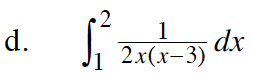Use partial fraction decomposition.

$\frac{1}{2x(x-3)}=\frac{a}{2x}+\frac{b}{x-3}$

$a(x-3)+b(2x)=1$

$-3a=1\text{ and }ax+2bx=0x$

$a=-\frac{1}{3}\text{ }b=\frac{1}{6}$

$\int_1^2\Big(-\frac{1}{6x}+\frac{1}{6(x-3)}\Big)dx$